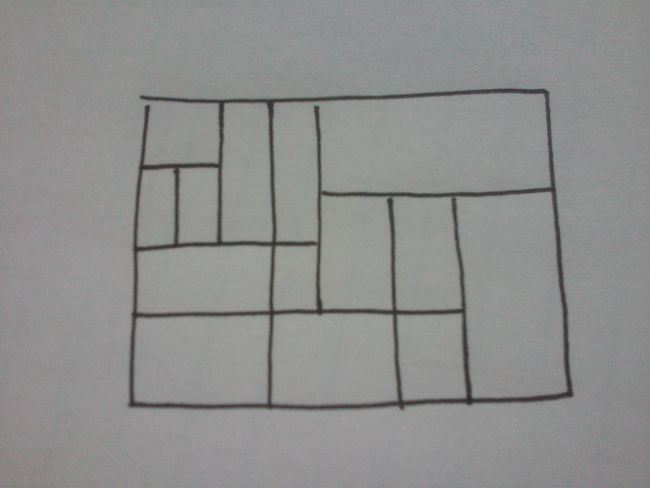# Cut Table

Logic Level 3A rectangular table has been cut into subrectangles whose edges are parallel to the original table, as in the example above. Given that each subrectangle has at least one side of integer length (in centimeters), is it true that the original table has at least one side of integer length (in centimeters)?

×